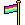kasploosh.com

# Decimal Time

"Decimal time" is another concept that is related to the Mike Date. The Mike Date is an example of decimal time.

## What Is Decimal Time?

Decimal time is using powers of ten to measure time. There are many different ideas about how to implement decimal time, and some of the ideas are quite old. A form of decimal time was used during the French Revolution.

Decimal time can be contrasted with "standard time" which most people use these days. Decimal time uses the base number 10 for most things. Standard time uses series of 2, 7, 10, 12, 28, 29, 30, and 31.

## Examples

One common implementation of decimal time is the Julian Date (and all its variations). The Julian Date uses a count of days since a specified starting point, with time of day represented as decimalized days. Astronomers use different flavors of the Julian Date.

Another implementation of decimal time is Unix Time, which takes a different approach. Unix Time is a neverending count of seconds since a certain starting point.

Computers and astronomers have moved to decimal time for similar reason—decimal numbers are easier to work with and easier to share with others.

It makes sense to have a measuring system based on tens, when your counting system is based on tens. The metric system is a good example of this idea.